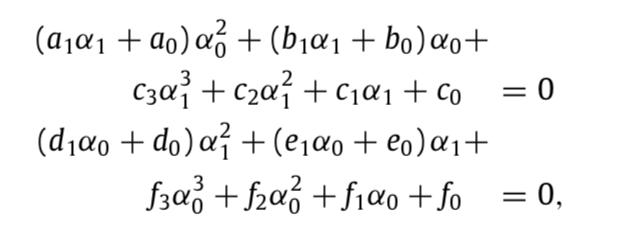# Smooth transitions between curve pieces in R^n

Hi
One of the innovative features in OpenCN is our approach how smooth transitions between curve pieces are computed.
The approach is based on “Optimal Hermite” interpolation with geometric continuity G^2.
Refer to our publication

This initially only worked for curves in R^3.

But it turns out that it is quite simple to generalize our concept for curves in R^n.

This is work in progress and will be a cornerstone of our E2C project aiming at 5 axes support of OpenCN.

best regards

1 Like

Great!

So the five axes coordinate is described in R^5 space instead of position(R^3) + 3d unit axis or position(R^3) + quaternion(SO(3)) space?

Could this method also control the axis orientation within a specified error?

The generalization for the computation of transitions is based on the observation that the notion of curvature vector can be defined for curves in R^n without need of “cross product” in R^3.

We will introduce in the cost function a diagonal weighting matrix allowing to weight cartesian coordinates x, y, z against rotation angles, e.g. B and C.

The error between the programmed path (G code) and the transition is given by the distance between the “lift-off” points and the discontinuity point where the curve pieces intersect.

Refer to our publication.

Just for curiosity. How is the curvature vector described in R^n?
Do you have an ongoing branch for this feature? Thanks~

Hi Yakunix,

Courbes de transition.pdf (28.0 KB)

prepared by Philippe Blanc.
Il explains the generalization of the curvature vector in R^n, and the generalization of our approach for generating smooth transitions between curve pieces.

There will be a specific branch.
At my knowledge, the function
G2_Hermite_Interpolation.m
has already be changed for 5 axes.

1 Like

Thank you Raoul. I will study the material and the code.

Hi Raoul, I checked the function here:

Seems not get updated. There are still cross product in the function CalcFrenet.m.

The last two equations in the material does not contain the curvature k, which exists in the original paper. Is it a mistake?

Yes, the scalar parameter kappa no longer appears since it is integrated in curvature vector n !

Be careful : BEFORE, vector n was the normal unit vector of the Frenet frame.
NOW, the new curvature vector is kappa times the old one.

To avoid misunderstandings, we renamed the curvature vector in n_k.
So, n_k = kappa * n

I will send you the link to the new branch.

1 Like

OK I see. So the coefficients of the polynomial system remains the same except the change of the calculation of the vector n.
I think it may also be useful for trajectory planning for manipulator in joint space R^n, where we have to create transitions between adjacent linear segments. Will give it a try.
Thanks!

we almost finished
G2_Hermite_Interpolation.m
for 5 axes.

G2_Hermite_Interpolation.m calls some functions created from symbolic calculations done in
https://gitlab.com/mecatronyx/opencnc/optimal-g2-hermite-interpolation-for-3d-curves/-/blob/master/Symbolic_Calculations.m

We modified Symbolic_Calculations.m for n = 5.
Unfortunately symbolic calculations need to know n in advance.

We’ll keep you updated at soon as the new code for transitions is ready and tested.

1 Like

Cool, Look forward to seeing it!

Hi @Raoul, I found the transition creation has been updated in the opencn repo. But I did not find the matlab function to generate code from symbolic expression. For example, where is the symbolic expression for the function CoefPolySys? Thanks!

I think the code is generated from the same equation as the 3-d case?Do you have any guide to select the weighting matrix A?

Our machine has a cartesian working space of ~50 x 50 x 50 mm.
The angles of the B and C axes are in degree, which is a comparable order of magnitiude. So the choice of our A matrix is identity.

We introduced A for future use where the cartesian and the rotary coordinates are not in a comparable order of magnitude.

Concerning the files for symbolic calculations, Hugo will reply you soon.

A “guide” for the choice of matrix A would be to choose a diagonal matrix with scaling factors enabling to scale all maximum excursions of cartesian and rotary coordinates to the normalized interval [-1, +1].

Thank you Raoul. I just modified the original symbolic script. It seems we can just remove the kappa param and resize the input vector. One thing I noticed is with the dimension increased from 3 to 5, the generated CoefPolySys.m will have more code (number of line increased from 257 to 1818). If I increased the dimension to 7, the generated file will have over 10000 line. Is it an expected behavior?hi Yakunix,
in principle, the smooth transitions could be coded in such a way, that n is specified at run time and not in advance, and the number of Matlab lines would be independant on n.
Observe that a lot of scalar products between vectors occur in the calculations.
Unfortunately, the symbolic math toolbox breaks these scalar product up, i.e.
instead of x’ times y, you find x1 times y1+x2 times y2+x3 times y3+ etc etc
And during the symbolic calculations, the expressions become more and more complicated.
The Matlab symbolic toolbox is not able to handle symbolic vectors with unspecified size.
I see two possibilities :

1. doing the calculations by hand instead by the symbolic toolbox2. with subs command, substitute scalar products by a new symbolic temporary variable

Thanks for pointing out this complexity issue.

OK, I see.
Thanks for your suggestion. Will give it a try.

Hello,

We added the script to generate the Matlab functions from the symbolic expression used in our algorithm for G2 smooth transition.

The latest version is now in the master in Feedopt/transition sub directory. The code generation has been tested and validated for 3 and 5 axes.

Matlab file : Symbolic_Calculations_nD.m

1 Like